Next: Interval Arithmetic Up: Approaches to Real Arithmetic Previous: Floating Point Arithmetic

## Floating Point arithmetic with error analysis

One approach to dealing with the problems of accuracy when using floating point arithmetic is to perform error analysis. This involves doing calculations to obtain a bound on the error of a particular expression.

One approach is to use the assumption that a real number x is approximated by the number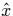, where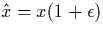. In this equation,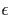is the relative error in the representation. Hence: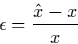It is now possible to calculate the effect that certain operations will have on the relative error of a floating point computation. Operations such as floating point multiplication will affect the relative error, but not significantly. Let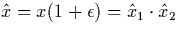where the desired result is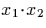:

Operations such as addition or subtraction, however, can have a much more significant effect on the relative error in certain cases. Consider the subtraction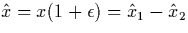:

It is now clear that if x2 is nearly equal to x1, the relative error will be greatly magnified.

The use of simple methods like this, or more sophisticated approaches, allow the accuracy of a given computation to be examined. This may allow the user to have faith in the results of a floating point computation. Knuth  describes the approach illustrated here and gives a more detailed discussion of the problem.

The major problems with such methods are that firstly the error analysis may simply tell the user that he or she should have no faith whatsoever in the correctness of the result produced, and secondly that the error analysis must be performed for every computation and is not general.Next: Interval Arithmetic Up: Approaches to Real Arithmetic Previous: Floating Point Arithmetic
Martin Escardo
5/11/2000